# Problem 52120. Compute the fractional derivative

Cody Problem 1370 asks us to compute the derivative of a polynomial. This problem extends that idea to fractional derivatives, which appear in some models of mixing in rivers and other applications. Denote the qth derivative as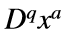. Then a familiar example from calculus would be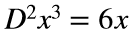.
Fractional calculus involves derivatives in which the order q is not an integer. With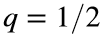and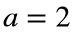, thenWrite a function that computes the fractional derivative of order q of an expression of the form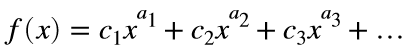The first input to the function will be a 2xn matrix in which the first row is the coefficients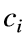and the second row is the exponents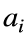. The output should be in a similar form.

### Solution Stats

35.29% Correct | 64.71% Incorrect
Last Solution submitted on Nov 16, 2022

### Community Treasure Hunt

Find the treasures in MATLAB Central and discover how the community can help you!

Start Hunting!